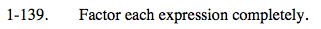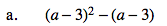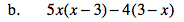### Home > PC > Chapter 1 > Lesson 1.4.1 > Problem1-139

1-139.
1. Factor each expression completely. Homework Help ✎

1. (a − 3)2 − (a − 3)

2. 5x(x − 3) − 4(3 − x)Factor out common terms or expressions.

(a − 3) is common to both terms.
So: (a − 3)((a − 3) − 1) = (a − 3)(a − 4)Follow the same procedure as (a).